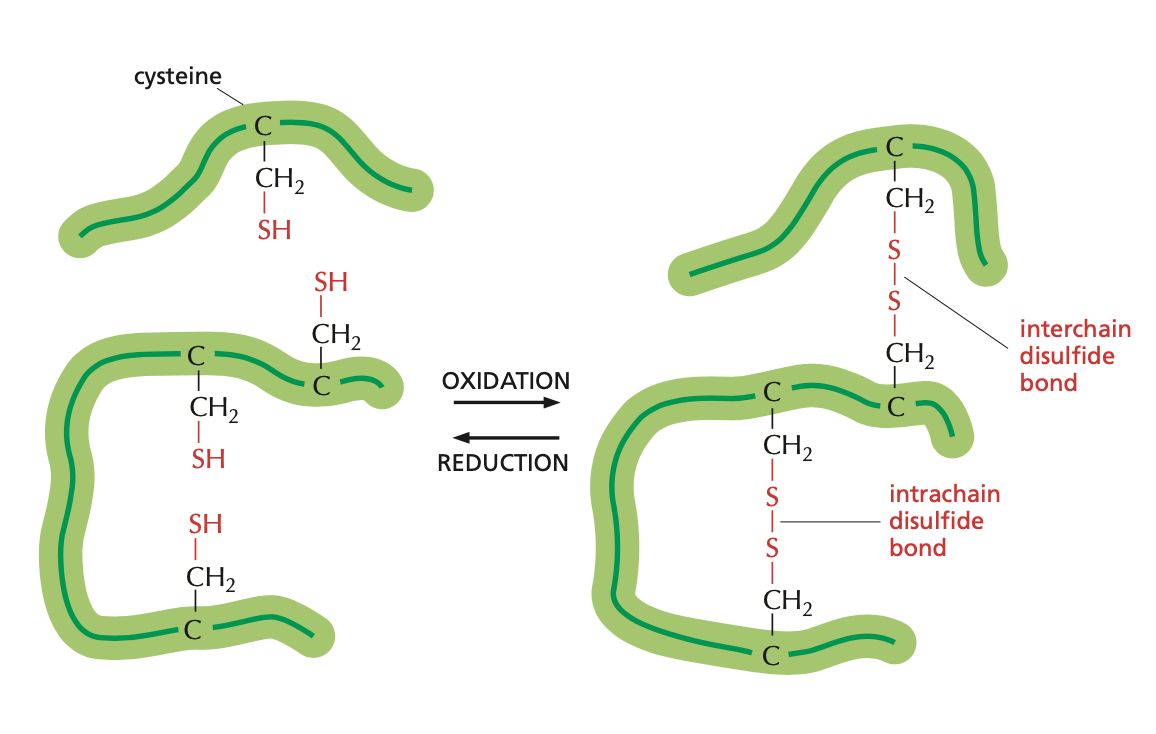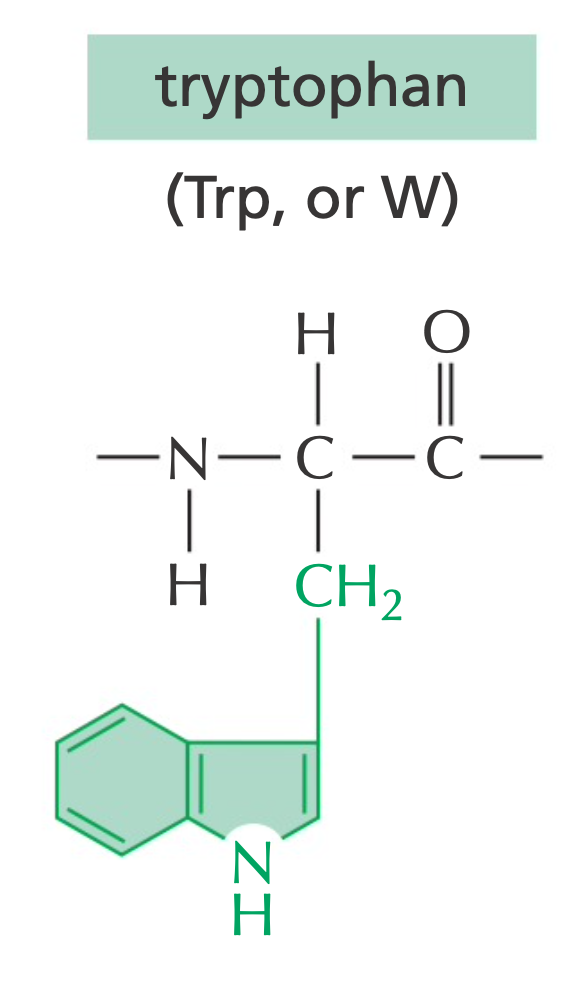## Constructing PAM1 Matrix

First, we define the frequency $$f(j)$$ and relative mutability $$m(j)$$ of a given animo acid j as:

$$f(j)=\frac{n(j)}{N}$$

where $$n(j)$$ is the number of occurrences of the $$j$$th amino acid, and $$N$$ is the total number of amino acids (20).

$$m(j)=\frac{\sum_{i}{A_{ij}}}{n(j)}$$

where $$A_{ij}$$ is the element from the accepted point mutation matrix shown above. The data in the matrix is calculated from a phylogenetic analysis where Dayhoff et al. empirically observed amino acid substitutions among aligned homologous sequences.

We then proceed to calculate the elements in the mutation probability matrix $$M_{ij}$$

$$M(i,j)=\frac{\lambda m(j)A_{ij}}{\sum_{i,i\neq j}^{20} A_{ij}}=\frac{\lambda A_{ij}}{Nf(j)}$$

For diagonal elements,

$$M(j,j)=1-\lambda m(j)$$

$$\lambda$$ is a constant of proportionality which is chosen so that in a PAM1 matrix

$$0.99=1-\lambda \sum_{j=1}^{20}{f(j)m(j)}$$

These calculations will give us the PAM1 mutation probability matrix.

The PAM scoring matrix is defined as a relatedness odds matrix, so in order to get the actual PAM scoring matrix, we need to convert the probability values into odds.

💡 Probability v.s. Odds: The probability of an event is the number of ways that an event can occur divided by the total number of possible outcomes. The odds for an event is the ratio of the number of ways the event can occur to the number of ways it does not occur.

$$\rm{odds}=\frac{probability}{1-probability}$$

The odds of an authentic amino acid match is defined as

$$R(i,j)=\frac{M(i,j)}{f(i)}$$

Finally, we can calculate the score of each mutation by scaling up the odds ratio using an logarithm.

$$s(i,j)=10\times\log_{10}{\frac{M(i,j)}{f(i)}}$$

## From PAM1 to PAM250

The evolution of DNA/amino acid sequences can be defined with a Markov chain model.

The definition of Markov chain model is:

A discrete stochastic (random) process X1, X2, X3, ... which has the Markov property so that

$$P(X_{n+1}|X_1=x_1,X_2=x_2,...,X_n=x_n)=P(X_{n+1}|X_n=x_n)$$

In another word,

A random process which has the property that the future (next state) is conditionally independent of the past given the present (current state).

Let's say that the probability of base/amino acid at a given position in stage n is vector $$q_n$$. Then, we have:

$$q_{n+1}=q_nP$$

where $$P$$ is the mutation probability matrix.

In stage $$n+k$$, we have:

$$q_{n+k}=q_nP^k$$

This tells us that the mutation probability matrix is multiplicable.

The base unit of time for the PAM matrices is the time required for 1 mutation to occur per 100 amino acids, sometimes called 'a PAM unit' or 'a PAM' of time. In the derivation for the PAM1 matrix, $λ$ is chosen to correspond to an evolutionary distance of 1 PAM. A larger $λ$ will result in a greater evolutionary distance.

One of the most commonly used PAM matrices is PAM250, in which 250 mutations occur in two aligned sequences of length 100. PAM250 is derived by multiplying the underlying mutation probability matrix $M$ 250 times before converting it to a log-odds matrix.

$$M_n=M_1^n$$

$$s_n(i,j)=10\times PAM_n(i,j)=10\times\log_{10}{\frac{M^n(i,j)}{f(i)}}$$

Therefore,

$$s_{250}(i,j)=10\times\log_{10}{\frac{M^{250}(i,j)}{f(i)}}$$

## Some Observations From PAM Matrices

By looking at the mutation probability matrix, we can tell that some amino acids are less mutable than others, namely Cysteine (C) and Tryptophan (W). And some small, polar amino acids are more acceptably mutable than others.

The side chains for cysteine and tryptophan have less common structures. Cysteine's side chain contains sulfur that can form disulfide bonds with other cysteine molecules, which is often important to a protein's tertiary structure.Formation of disulfide bond from the cysteine residue

Tryptophan is less mutable because of its large aromatic side chain.Structure of tryptophan with the large aromatic ring highlighted

## BLOSUM Matrix

Created by Steven Henikoff and Jorja Henikoff, the BLOSUM62 is another commonly used substitution matrix and the default scoring matrix for NCBI BLASTP (Protein BLAST). The BLOSUM62 matrix is designed to find conserved regions of proteins.

The BLOSUM matrix is calculated from the BLOCKS database, which contains large number of local alignments of conserved regions of distantly related proteins. The sequences are clustered based on their percent identity.

Unlike PAM, the BLOCKS alignments used to derive the BLOSUM matrices include sequences that are much less similar to each other, but whose evolutionary homology can be confirmed through intermediate sequences. In addition, these alignments are analyzed without creating a phylogenetic tree and are simply compared with each other.

To start with, we calculate the observed probability from the aligned sequences.

$$q(i,j)=\frac{f(i,j)}{\sum_{i=1}^{20}\sum_{j=1}^jf(i,j)}$$

where $$f(i, j)$$ is the weighted frequency (number) of $$i$$ aligning with $$j$$. The denominator is the total number of aligned pairs.

The score can be obtained using this equation

$$s(i,j)=2\log_2\left(\frac{q(i,j)}{p(i,j)}\right)$$

where $$p(i, j)$$ is the background probability (the probability of i and j aligned by chance instead of actual homology).

In general terms,

$$s(i,j)=\frac{1}{\lambda}\ln\left(\frac{q(i,j)}{p(i)p(j)}\right)$$

Below is the BLOSUM62 matrix with a identity threshold of 62%, which means during the process of constructing the matrix, sequences with identity ≥62% are merged into a cluster.

## PAM v.s. BLOSUM

Equivalency

### Differences

PAM

• Used to score closely related sequences
• Based on global alignments
• High similarity
• Calculated based on evolutionary distance

BLOSUM

• Used to score distantly related sequences
• Based on local alignments
• Low similarity
• Calculated based on sequence similarities

## Reference

Construction of substitution matrices

Point accepted mutation

BLOSUM

Alberts, B., Johnson, A., Lewis, J., Morgan, D., Raff, M. C., Roberts, K., . . . Hunt, T. (2015). Molecular biology of the cell. New York, NY: Garland Science.

Pevsner, J. (2016). Bioinformatics and Functional Genomics. Chichester, West Sussex: Wiley Blackwell.

Zvelebil, M. J., & Baum, J. O. (2008). Understanding Bioinformatics. New York: Garland Science/Taylor & Francis Group.